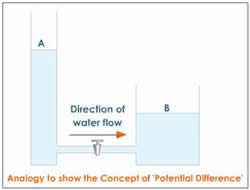# Difference between Voltage and Potential Difference

##### Key Difference: Voltage is the electric potential between two different points. It could also be used to refer to the difference in electric potential energy of a unit test charge transported between two points. Electric potential difference is the same as voltage.There are many words in the English language that have many different terms referring to the same thing, depending on the scenario it is used in. These many different terms often create confusing among people that are no well-versed with the field. Voltage and Potential difference are two words that refer to the same thing, a difference between two points.

Voltage is the electric potential between two different points. It could also be used to refer to the difference in electric potential energy of a unit test charge transported between two points. A voltage may represent a source of energy or it could represent lost, used or stored energy. Voltage is the pressure of pushing electrons along in a circuit. Two paths require voltage in order for a current to pass through them. Voltage is also the total energy required to move a small electric charge between two points. Voltage is defined in such a way that negatively charged objects are pulled towards higher voltages, while positively charged voltages are pulled towards lower voltages. Volts are measured using a voltmeter.

Electric potential difference is defined by The Free Dictionary as, “The amount of energy per unit charge needed to move a charged particle from a reference point to a designated point in a static electric field.” This is the same as voltage. There is essentially no difference between the two words.

Image Courtesy: tutorvista.com